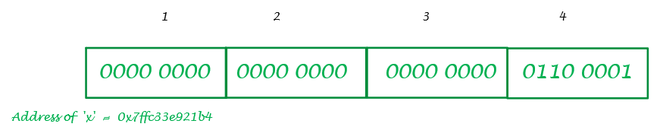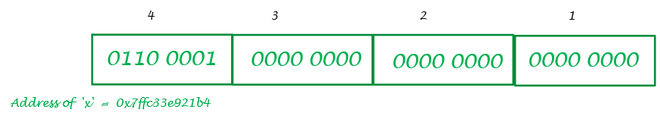Open in App
Not now

# Understanding Typecasting as a compiler

• Last Updated : 17 Sep, 2021

Let’s start our discussion with a very few lines of C –

## C

 `#include ` ` `  `int` `main() { ` ` `  `    ``int` `x = 97; ` `    ``char` `ch = x; ` `    ``printf``(``"The value of %d in character form is '%c'"``,x, ch); ` `    ``return` `0; ` `} `

Output :

`The value of 97 in character form is 'a'`

Let us understand that how the compiler is going to compile every instruction in this program.

Compiler :

1. Enters the main program
2. Allocated  4 bytes of memory ( as integer takes 4 bytes generally), named it as x, and gives it a value of 97
3. Allocated 1 byte of memory ( as a character takes 1 byte of space ), named it as ch, and stored the value corresponding to x in it
4. Printed the value of ch
5. Exits the main program

We will understand 2 & 3 points thoroughly as all others are obvious.

Description of the second point :

The compiler will convert 97 ( in decimal ) to its corresponding binary form as the memory stores the data in the binary form. 00000000 00000000 00000000 01100001 is the binary representation of 97 in 4-byte(or 32-bit) binary representation. So the content of the variable x is going to look like as in the given image –Big Endian System

This system of representation of the data in binary form in the memory is known as Big Endian But in most computer systems despite this system, the Little Endian system is followed. So in the Little Endian system content of x is as below –Little Endian system

So in most computers, every data in the memory is going to be stored in the Little-Endian form only.

Description of the second point :

As said earlier the character takes up 1 byte or 8 bits of space in the memory so the compiler will allocate that but when it comes to assigning the value to the variable ch it will go at the location where x is present and start reading the content of only first byte as it is reading for a character. So the first byte has 0110 0001 in binary form and its corresponding decimal form is 97. Every character has some ASCII code associated with it and the ASCII code for character is 97. So that is why the content of ch will become a and after that, the compiler will simply print the content of ch.

Some more examples for better understanding :

## C

 `#include ` ` `  `int` `main() { ` `    ``int` `x = 321;  ``//can be written as 256+65 ` `    ``char` `ch = x; ` `    ``printf``(``"The character form of %d is '%c'"``,x,ch); ` `    ``return` `0; ` `} `

Output :

`The character form of 321 is 'A'`

Explanation :

The binary representation of 321 is 00000000 00000000 00000001 01000001 and the content of x in Little Endian system is 0100001 00000001 00000000 00000000 simply do 1234 ⇢ 4321 (see image) do get this form. Now the compiler only looks only on the first byte which is  0100001 and its corresponding decimal form is 65 which is the ASCII code for character ‘a’.

## C

 `#include ` ` `  `int` `main() { ` ` `  `    ``int` `x = 57;  ` `    ``char` `ch = x; ` `    ``printf``(``"The character form of %d is '%c'"``,x,ch); ` `    ``return` `0; ` `} `

Output :

`The character form of 57 is '9'`

Explanation :

The binary representation of 57 is 00000000 00000000 00000000 00111001 and the content of x in Little Endian system is 00111001 00000000 00000000 00000000 Now the compiler only looks only on the first byte which is 00111001 and its corresponding decimal form is  57 which is the ASCII code for character ‘9’.

Nice To Have :

1. ASCII code for A-Z is 65-90, a-z is 97-122, and digits from 0 to 9 also have their corresponding ASCII code from 48 to 57.
2. To find the corresponding character form simply find the modulo of that number with 256 and then look up the ASCII table and find the character corresponding to the remainder. That will be your answer.

THANKS  ^_^

My Personal Notes arrow_drop_up
Related Articles